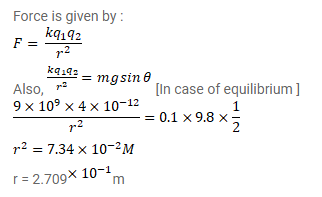# A particle having a charge ofQuestion:

A particle having a charge of $2.0^{\times 10^{-6}} \mathrm{C}$ and a mass of $100 \mathrm{~g}$ is placed at tom of a smooth inclined plane of inclination $30^{\circ}$ Where should another particle B, having same charge and mass, be placed on the incline so that it may remain in equilibrium?

Solution: